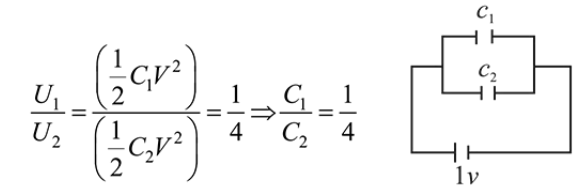# Effective capacitance of parallel combination of two capacitorsQuestion:

Effective capacitance of parallel combination of two capacitors $C_{1}$ and $C_{2}$ is $10 \mu \mathrm{F}$. When these capacitors are individually connected to a voltage source of $1 \mathrm{~V}$, the energy stored in the capacitor $C_{2}$ is 4 times that of $C_{1}$. If these capacitors are connected in series, their effective capacitance will be:

1. (1) $4.2 \mu \mathrm{F}$

2. (2) $3.2 \mu \mathrm{F}$

3. (3) $1.6 \mu \mathrm{F}$

4. (4) $8.4 \mu \mathrm{F}$

Correct Option: , 3

Solution:

(3) In parallel combination, $C_{\text {eq }}=C_{1}+C_{2}=10 \mu F$ When connected across $1 V$ battery, then$\therefore C_{2}=8 \mu F$ and $C_{1}=2 \mu F$

Now $C_{1}$ and $C_{2}$ are connected in series combination,

$\therefore C_{\text {equivalent }}=\frac{C_{1} C_{2}}{C_{1}+C_{2}}=\frac{2 \times 8}{2+8}=\frac{16}{10}=1.6 \mu F$